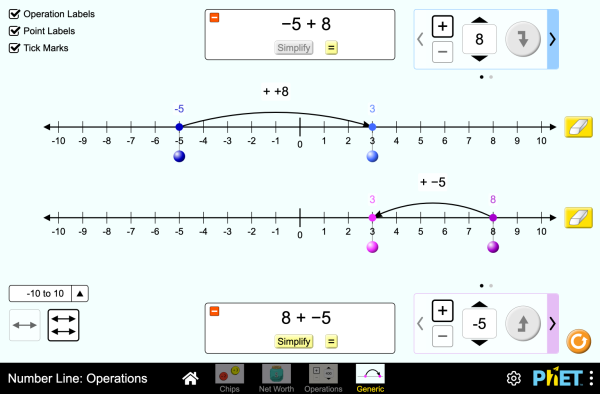# Number Line: Operations

Tato simulace dosud nebyla přeložena do tohoto jazyka. Prozatím použijte anglickou verzi.StáhnoutSdílet zavřít Použít vloženou (embedded) kopii této simulace Použijte tento HTML kód k vytvoření vložené kopie této simulace. Můžete změnit výšku a šířku vložené kopie změnou atributů "width" a "height" v HTML kódu. Vnořit obrázek, který při kliknutí spustí simulaci
Klikni pro spuštění
Použijte tento HTML kód k zobrazení náhledu simulace se slovy "Klikni pro spuštění". Operations Number Line Integers PhET je podporován:a učiteli jako vy.

• Operations
• Number Line
• Integers

### Description

Explore operations with integers using an authentic context of net worth, then generalize with a number line. Experiment with adding and subtracting both positives and negatives, and make predictions about whether the total will be positive or negative.

• Represent addition and subtraction of integers on a horizontal number line
• Reason about addition and subtraction of integers in terms of number-locations
• Use logical necessity to reason that addition has the opposite effect as subtraction or that adding (or subtracting) a negative integer has the opposite effect as adding (or subtracting) a positive integer.
• Recognize and generate equivalence classes of integer sums and differences
• Show that a number and its additive inverse (opposite) have a sum of 0
• Using a net worth context, describe situations that have a positive sum or difference, negative sum or difference, and zero sum or difference
• View adding a negative as equivalent to subtracting a positive, and explain why this relationship makes sense in contexts such as net worth.
• View subtracting a negative as equivalent to adding a positive, and explain why this relationship makes sense in contexts such as net worth.
• Apply a number line model for addition and subtraction to new contexts.

### Standards Alignment

#### Common Core - Math

7.NS.A.1
Apply and extend previous understandings of addition and subtraction to add and subtract rational numbers; represent addition and subtraction on a horizontal or vertical number line diagram.
7.NS.A.1a
Describe situations in which opposite quantities combine to make 0. For example, a hydrogen atom has 0 charge because its two constituents are oppositely charged.
7.NS.A.1b
Understand p + q as the number located a distance |q| from p, in the positive or negative direction depending on whether q is positive or negative. Show that a number and its opposite have a sum of 0 (are additive inverses). Interpret sums of rational numbers by describing real-world contexts.
7.NS.A.1c
Understand subtraction of rational numbers as adding the additive inverse, p - q = p + (-q). Show that the distance between two rational numbers on the number line is the absolute value of their difference, and apply this principle in real-world contexts.
7.NS.A.1d
Apply properties of operations as strategies to add and subtract rational numbers.
Version 1.0.1

### Teacher TipsUčitelská příručka (pdf) obsahuje tipy poskytnuté od členů týmu PhET. ( PDF ).

### Teacher-Submitted Activities

Integer Addition and Subtraction Assessment Gillian Walker ZŠ II.st.
Lab
Remote
Matematika
Basque All EuskaraZenbaki-lerroa: eragiketak
Bosnian All BosanskiBrojevna prava: Operacije
Croatian All hrvatskiBrojevna crta: Računske operacije
Danish All DanskTallinje: Beregninger
Dutch All NederlandsGetallijn: Bewerkingen
French All françaisDroite numérique: opérations
German All DeutschZahlenstrahl: Operationen
Greek All ΕλληνικάΑριθμογραμμή: πράξεις
Hungarian All magyarSzámegyenes - műveletek
Chinese (Taiwan) All 中文 (台灣)Number Line: Operations_數線：數線的運算
Italian All italianoRetta dei numeri: operazioni
Latvian All LatviešuSkaitļu ass: Darbības
Maori All MaoriRārangi Tau: Paheko
Polish All polskiOś liczbowa: działania
Portuguese (Brazil) All português (Brasil)Reta Numérica: Operações
Serbian All СрпскиБројевна права: Операције
Slovak All SlovenčinaČíselná os: Operácie
Spanish All españolRecta Numérica: OperacionesSpanish (Peru) All español (Perú)Recta numérica: Operaciones
Turkish All TürkçeSayı Doğrusu: İşlemler
Vietnamese All Tiếng ViệtĐường thẳng số: Các phép toán

HTML5 sims can run on iPads and Chromebooks, as well as PC, Mac, and Linux systems on modern web browsers. If you are experiencing issues using an HTML5 sim on a supported platform, please disable any browser extensions.

iOS 12+ Safari

Android:
Not officially supported. If you are using the HTML5 sims on Android, we recommend using the latest version of Google Chrome.

Chromebook:
The HTML5 and Flash PhET sims are supported on all Chromebooks.
Chromebook compatible sims

Windows Systems: